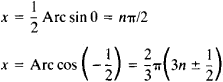# Trigonometric Equation

The following article is from The Great Soviet Encyclopedia (1979). It might be outdated or ideologically biased.

## Trigonometric Equation

an algebraic equation involving trigonometric functions of the variables. To solve a trigonometric equation by using different relations among trigonometric functions, the trigonometric equation is converted to a form such that the values of one of the trigonometric functions of the desired variable can be determined. The roots of the trigonometric equation are then found by means of inverse trigonometric functions. For example, sin x + sin 2x + sin 3x = 0 can be reduced to the form 2 sin 2x cos x + sin 2x = 0 or sin 2x (2 cos x + 1) = 0; thus, sin 2x = 0 or cos x = – 1/2. This result gives the solutions of the trigonometric equationwhere n is an arbitrary positive or negative integer.

References in periodicals archive ?
The angle was calculated from measurements done with a metric tape ([+ o -] 1 mm) of the height of the heap at two points and the horizontal length between them, applying the trigonometric equation:
From the historical perspective, in this process of development and expansion, for equations, the linear equation, dual linear equation, quadratic equation, multiple equation, geometry equation, trigonometric equation, ordinary differential equation, partial differential equation, integral equation, and the like are appeared step by step; for solutions, the approximate solution, accurate solution, analytical solution, numerical solution, and the like are also appeared step by step.
Biquadratic trigonometric equation 2 [tan.sup.4] 3x - 3 [tan.sup.2] 3x + 1 = 0 is too complicated for Maxima and Sage, but 2[t.sup.4] - 3[t.sup.2] + 1 = 0 is solvable.
The first approach is based on a basic trigonometric equation
The solution for the trigonometric equation (1) is:
Four areas are discussed more thoroughly - multiplicity of roots, extraneous roots, literal equation and trigonometric equation.
This model is suitable to implement trigonometric equations in FPGA devices.
* This method can be used to solve trigonometric equations.
I'M sure wind power enthusiasts Trefor Davies and Peter Leach (Letters) would never put pen to paper, advising mathematicians on how to carry out complex vector or trigonometric equations.
Physicists have complex trigonometric equations, known as the Helmholtz Function and the
Even though mathematics education in Australia varies considerably between various States due to the different education systems in place in each State, the trigonometric functions and subsequently solving trigonometric equations including the periodicity of those functions are included in the various levels of mathematics learned in Years 11-12.
This two trigonometric equations system are compatible according to a and [beta] variables.
Site: Follow: Share:
Open / Close